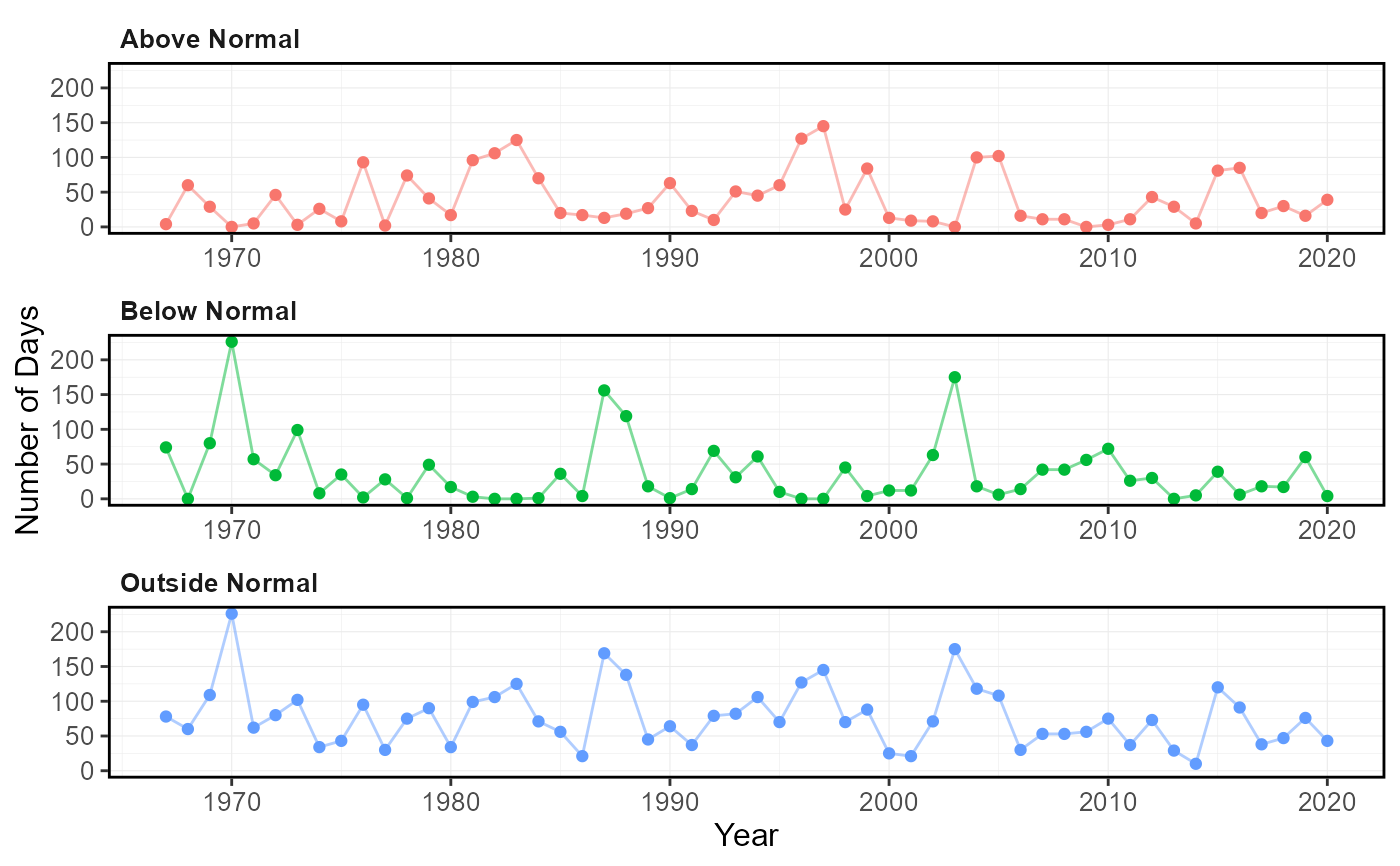Plots the number of days per year outside of the 'normal' range (typically between 25 and 75th percentiles) for each day of the year. Upper and lower-range percentiles are calculated for each day of the year of from all years, and then each daily flow value for each year is compared. All days above or below the normal range are included. Calculates statistics from all values from complete years, unless specified. Data calculated using calc_annual_outside_normal() function. Returns a list of plots.

plot_annual_outside_normal(
data,
dates = Date,
values = Value,
groups = STATION_NUMBER,
station_number,
normal_percentiles = c(25, 75),
roll_days = 1,
roll_align = "right",
water_year_start = 1,
start_year,
end_year,
exclude_years,
months = 1:12,
include_title = FALSE
)

## Arguments

data Data frame of daily data that contains columns of dates, flow values, and (optional) groups (e.g. station numbers). Leave blank if using station_number argument. Name of column in data that contains dates formatted YYYY-MM-DD. Only required if dates column name is not 'Date' (default). Leave blank if using station_number argument. Name of column in data that contains numeric flow values, in units of cubic metres per second. Only required if values column name is not 'Value' (default). Leave blank if using station_number argument. Name of column in data that contains unique identifiers for different data sets, if applicable. Only required if groups column name is not 'STATION_NUMBER'. Function will automatically group by a column named 'STATION_NUMBER' if present. Remove the 'STATION_NUMBER' column beforehand to remove this grouping. Leave blank if using station_number argument. Character string vector of seven digit Water Survey of Canada station numbers (e.g. "08NM116") of which to extract daily streamflow data from a HYDAT database. Requires tidyhydat package and a HYDAT database. Leave blank if using data argument. Numeric vector of two values, lower and upper percentiles, respectively indicating the limits of the normal range. Default c(25,75). Numeric value of the number of days to apply a rolling mean. Default 1. Character string identifying the direction of the rolling mean from the specified date, either by the first ('left'), last ('right'), or middle ('center') day of the rolling n-day group of observations. Default 'right'. Numeric value indicating the month (1 through 12) of the start of water year for analysis. Default 1. Numeric value of the first year to consider for analysis. Leave blank to use the first year of the source data. Numeric value of the last year to consider for analysis. Leave blank to use the last year of the source data. Numeric vector of years to exclude from analysis. Leave blank to include all years. Numeric vector of months to include in analysis (e.g. 6:8 for Jun-Aug). Leave blank to summarize all months (default 1:12). Logical value to indicate adding the group/station number to the plot, if provided. Default FALSE.

## Value

A list of ggplot2 objects with the following for each station provided:

Annual_Days_Outside_Normal

a plot that contains the number of days outside normal

Default plots on each object:
Days_Below_Normal

number of days per year below the daily normal (default 25th percentile)

Days_Above_Normal

number of days per year above the daily normal (default 75th percentile)

Days_Outside_Normal

number of days per year below and above the daily normal (default 25/75th percentile)

## See also

calc_annual_outside_normal

## Examples

# Run if HYDAT database has been downloaded (using tidyhydat::download_hydat())
if (file.exists(tidyhydat::hy_downloaded_db())) {

# Plot annual statistics with default limits of normal (25 and 75th percentiles)
plot_annual_outside_normal(station_number = "08NM116")

# Plot annual statistics with custom limits of normal
plot_annual_outside_normal(station_number = "08NM116",
normal_percentiles = c(10,90))

}
#> Warning: One or more calculations included missing values and NA's were produced. Filter data for complete years or months, or use to ignore_missing = TRUE to ignore missing values.#> Warning: One or more calculations included missing values and NA's were produced. Filter data for complete years or months, or use to ignore_missing = TRUE to ignore missing values.#> \$Annual_Days_Outside_Normal#>